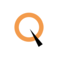A Guide to Power Function in Java

# A Guide to Power Function in Java

clickHere
Updated on Nov 23, 2023 14:54 IST

Have you ever wondered how mathematical power functions are implemented in programming languages like Java? In Java, the Math.pow() function is a powerful tool used to raise a number to a power. Let's understand more!

The Power Function is used to raise a number to the power of another number. It is a part of the Math class and can be used using Math.pow(double a, double b) where a is the base and b is the exponent. The method returns the result as a double.

Also, read Power Function in C++

## Syntax

double result = Math.pow(double base, double exponent);

Where,

• base is the number to be raised.
• exponent is the power to which the base number is raised.

This method is part of the Math class in Java and is used to perform exponentiation.

Example of Power Function in Java

`public class Main {    public static void main(String[] args) {        double base = 3.0;        double exponent = 4.0;                double result = Math.pow(base, exponent);                System.out.println("Result: " + result);    }}Copy code`

Output

`Result: 81.0`

In the above example, 3.0 is raised to the power of 4.0, and the result 81.0 is printed to the console. Don’t forget to import the java.lang package to use the Math class, although in most cases, it’s imported by default since it’s a part of the core Java package.Conditional Statements in Java
The below article goes through the Conditional Statements in Java. It covers if, if-else, nested if-else, and switch statements with examples.Features of Java Programming Language
Java is one of the most popular and commonly used programming languages. It is widely recognized for its performance, platform independence, and security. We have tried to explain features of...read moreStrings in Java Explained
The below article goes through explaining and implementing Strings in Java with appropriate examples.

## Let’s See a Few More Examples to Understand the Concept

### Basic Level

Example 1

Calculating the square of a number

`public class Main {    public static void main(String[] args) {        double result = Math.pow(5.0, 2.0);        System.out.println("Result: " + result);    }}Copy code`

Output

`Result: 25.0`

Example 2

Calculating the cube of a number

`public class Main {    public static void main(String[] args) {        double result = Math.pow(21.0, 3.0);        System.out.println("Result: " + result);    }}Copy code`

Output

`Result: 9261.0`

### Intermediate Level

Example 3

Calculating the square root using Math.pow

`public class Main {    public static void main(String[] args) {        double result = Math.pow(64.0, 0.5);        System.out.println("Result: " + result);    }}Copy code`

Output

`Result: 8.0`

Example 4

Compound Interest Calculation

```public class Main {    public static void main(String[] args) {        double principal = 1000.0;        double rate = 7.0;         double time = 4.0;          double compoundInterest = principal * Math.pow((1 + rate/100), time);        System.out.println("Compound Interest: " + compoundInterest);    }}Copy code```

Output

`Compound Interest: 1310.7960100000003`

This output indicates that the compound interest accrued over 4 years with a 7% interest rate on a principal of 1000 units of currency is 310.7961 units of currency (with the total amount being 1310.7961 units of currency).

### Miscellaneous Level

Example 5

Population Growth Calculation (Exponential Growth)

In this example, we will calculate the projected population of a city after a certain number of years, given an annual growth rate. The formula for exponential growth is:

## P = P0.e(r.t)

Where:

• P = final population
• P0 = initial population
• r = annual growth rate (as a decimal)
• t = time in years
• e = base of the natural logarithm (approximately 2.71828)

```public class Main {    public static void main(String[] args) {        double initialPopulation = 500000.0;        double growthRate = 0.02;        double timeInYears = 10.0;         double finalPopulation = initialPopulation * Math.pow(Math.E, growthRate * timeInYears);        System.out.println("Projected Population: " + finalPopulation);     }}Copy code```

Output

`Projected Population: 610701.379080085`

This example showcases a real-world application of the Math.pow() method, where it’s used to calculate exponential growth, a concept frequently seen in biology (population growth), finance (compound interest), and many other fields.Power Function in C
Have you ever wondered how mathematical calculations involving exponents are handled in the C programming language? One of the key functions used for this purpose is the pow() function, which...read morePower Function in C++
Learn about the power function in C++ and how to use the pow() function to perform mathematical calculations in C++ programs. This article covers the power of a number, how...read moreNested If Else in Java | About, Syntax, Flowchart and Examples
Have you ever wondered how programs make decisions and adapt to different situations? The answer lies in the power of Nested If else statements. These statements allow us to control...read moreDo you know what makes the code more readable? Comments, as they provide valuable context and explanations about the code, making it easier for both the original developers and others...read moreStar Pattern Programs in Java
Pattern programs in Java are a type of problem that use nested loops to produce different patterns of numbers, stars (*), or other characters. In this blog we will dive...read moreNumber Pattern Programs in Java
Pattern programs in Java are a type of problem that use nested loops to produce different patterns of numbers, stars (*), or other characters. In this blog we will dive...read more

## Conclusion

The Power Function in Java, Math.pow() allows developers to compute exponential values with ease. Throughout this blog, we’ve demonstrated its utility in various scenarios, from simple numeric operations to complex calculations involving compound interest and exponential growth.

clickHere

I'm Esha Gupta, a B.Tech graduate in Computer Science & Engineering, specializing in Front End Web Dev. I've interned at GeeksforGeeks & Coding Minutes, fueling my passion for crafting appealing and functional digit... Read Full Bio

## Trending Technology CoursesProgramming in Java by NPTEL
NPTEL4.6Java Full Stack
Qspider4.6Object Oriented Programming in Java Specialization
Coursera5.0Starts 5 Dec

## Top Picks & New Arrivals